Latest Banking jobs   »

# Quantitative Aptitude Quiz For SIDBI Grade A 2022- 20th December

Q1. In a bag, there are 16 balls of three different colors i.e. red, blue and green. Number of red and blue balls is 9 and difference between red and green ball is 4 then find the probability of getting a ball of each color if three balls are picked at random?
(a) 5/28
(b) 4/27
(c) 7/36
(d) 8/35
(e) 9/40

Q2. Cost price of article A is Rs 600 more than that of B and selling price of A is Rs 1200 more than that of B. If difference between profit earned on selling these two articles is 13⅓% of the cost price of A then find profit earned on B if profit % earned on A is 33⅓%?
(a) Rs 800
(b) Rs 900
(c) Rs 840
(d) Rs 960
(e) Rs 1000

Q3. In 100 lit mixture of milk and water, milk is 68 lit more than that of water. When ‘a’ lit of milk is taken out and (a+15) lit of water is added, milk becomes 50% more than that of water. Find value of ‘a’?
(a) 20
(b) 15
(c) 18
(d) 12
(e) 16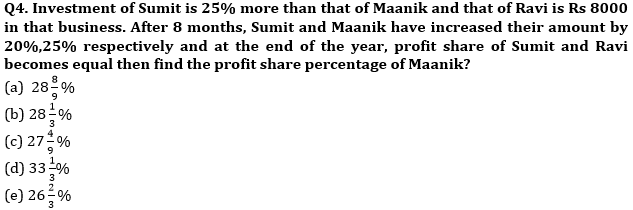Q5. Area of rectangle having length and breadth in the ratio of 4: 3 is 432 cm2. A square whose side is equal to diagonal of rectangle then find ratio of numerical value of perimeter to the area of square?
(a) 7:55
(b) 4:35
(c) None of these
(d) 1:8
(e) 2:15

Q6. Marked price of an article is Rs 360 more than its cost price. If profit earned is equal to the discount given then find the profit earned?
(a) Rs 190
(b) Rs 200
(c) Rs 150
(d) Rs 180
(e) Rs 175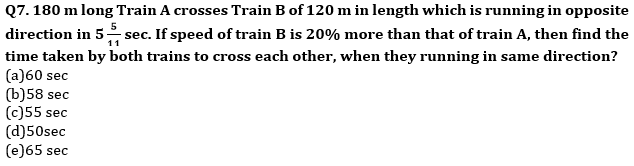Q8.Difference between CI received in first 1.5 years (compounded annually) at 20% per annum and CI received in last 1.5 years when compounded half yearly at the same rate of interest on the same sum is Rs 715 then find the sum?
(a) Rs. 66,000
(b) Rs. 65,000
(c) Rs. 64,500
(d) Rs. 65,500
(e) Rs. 67,500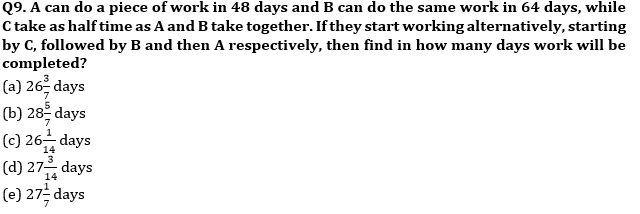Q10. Difference between downstream and upstream speed of a boat is 6 km/h. Find the total time taken by boat to cover 30 km in upstream if it takes 7 hours to cover a distance of 20 km both in downstream and upstream?
(a) 7 ½ hr
(b) 9 hr
(c) 8 hr
(d) 10 hr
(e) None of these

Q11. ‘A’ invested Rs. X on CI and ‘B’ invested Rs. Y on SI at the rate of 10% and 20% respectively. A invested for two years and B invested for three years and ratio of interest got by ‘A’ for second year and total interest got by B is 1 : 3. Veer invested Rs. (X + Y) on SI for two years on 12% p.a. and gets Rs. 372 as interest. Find (X – Y)?
(a) Rs. 350
(b) Rs. 450
(c) Rs. 400
(d) Rs. 750
(e) Rs. 800

Q12.Veer and Anurag invested Rs. 1200 & Rs. 1600 respectively and after 15 months Veer withdrew 2/23 th of total profit, while Anurag added 4/23 th of total profit. If at the end of two years share of profit ratio of Veer & Anurag is 9 : 14, then find the difference between profit share of Veer & Anurag?
(a) Rs. 400
(b) Rs. 450
(c) Rs. 500
(d) Rs. 550
(e) Rs. 600

Q13. Sum of ages of A and B is 12 years more than twice the age of C and Sum of ages of A & D is twice the age of C. If the average age of B&D is 50 years and average age of all four is also 50 years, then find the difference between the age of A and C?
(a) 6 years
(b) 1 years
(c) 3 years
(d) 4 years
(e) 2 years

Q14. Aman divided his total savings between his son and daughter in the ratio of 5 :3. His son invested his share on CI for two years at the rate of 10% and received the interest Rs. 480 less than his sister, who invested her share on SI for three years at the rate of 15% . Find Share of Aman’s son and daughter respectively?
(a) Rs. 10800, Rs. 2000
(b) Rs. 9600, Rs. 4200
(c) Rs. 8000, Rs. 4800
(d) Rs. 9800, Rs. 3000
(e) Rs. 7000, Rs. 5800

Q15. P and Q together can complete a work in 24 days, while Q and R working together can complete the same job in 32 days. P and Q started the work and did it for 8 days, after that P left the work and R joined Q and after 12 more days, Q also left the work. Then, the remaining work was completed by R in 28 days. Find in how many days R will complete the work alone?
(a) 96 days
(b) 72 days
(c) 108 days
(d) 90 days
(e) 81 days

Solutions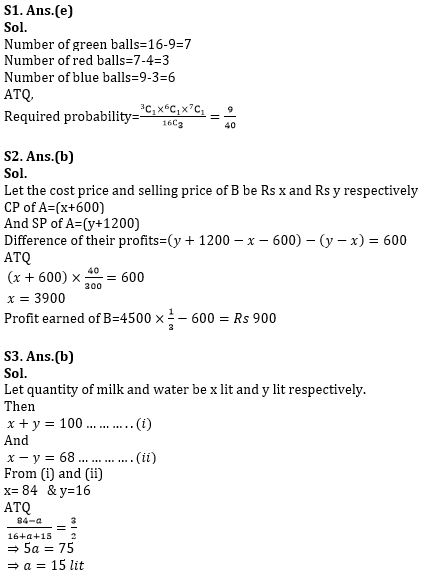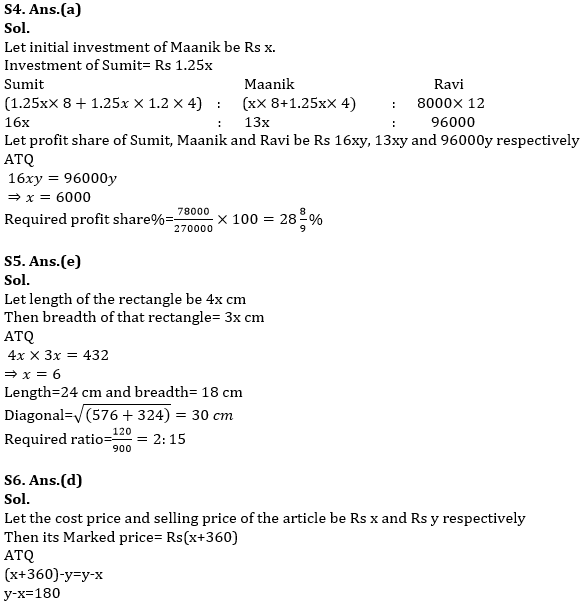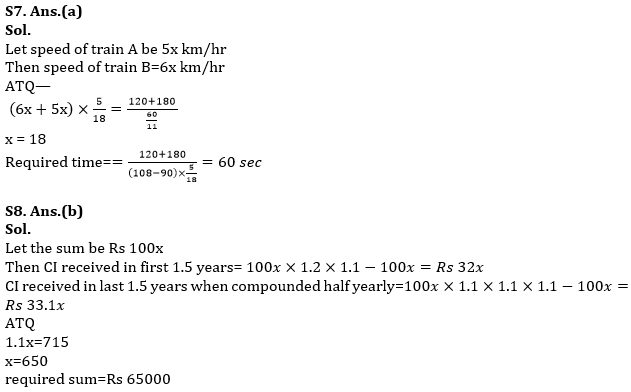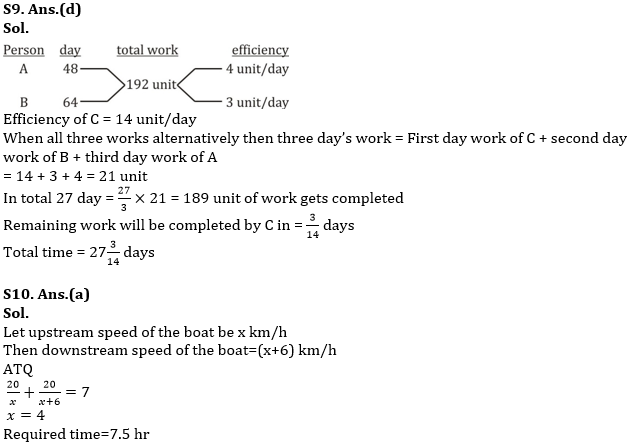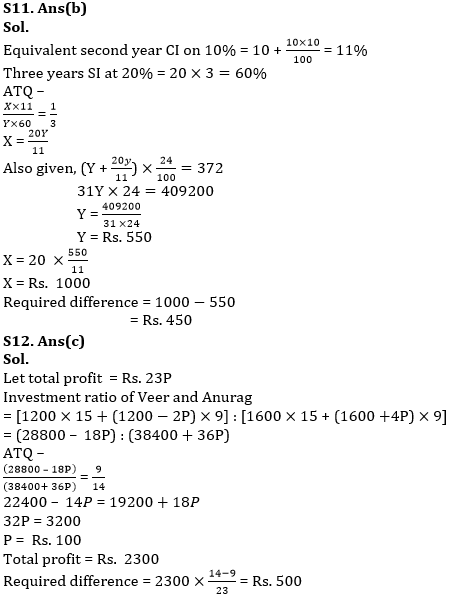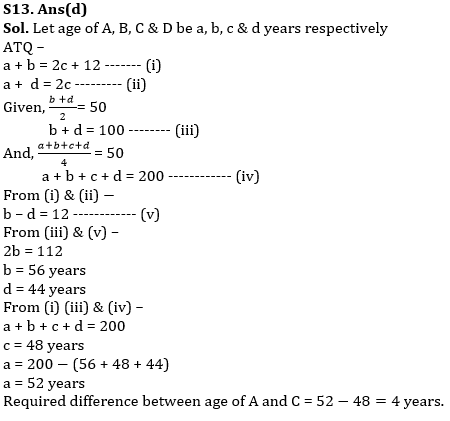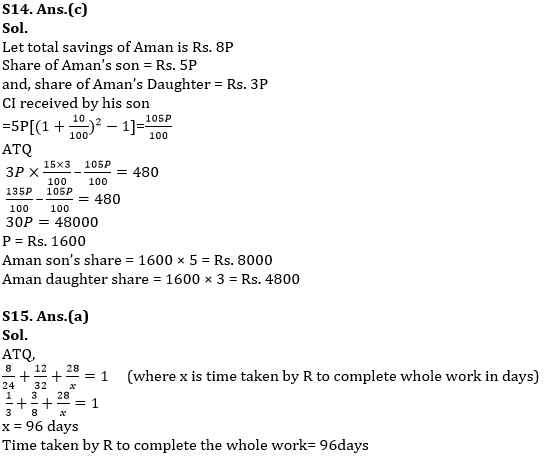#### Congratulations!Union Budget 2023-24: Free PDF